Operations & Maintenance

# Cooling Towers: Estimate Evaporation Loss and Makeup Water Requirements

| By U. Vengateson, National Petrochemical Co.

Applying mass and energy balance calculations yields critical operating insight

Cooling towers are an important unit operation in chemical process industries (CPI) operations. Applying mass and energy balance calculations enables process engineers to evaluate evaporation loss, blowdown and makeup-water requirements, and to evaluate the performance of the cooling tower. In this article, an illustrative study showcases an induced-draft cooling tower and describes several key parameters — range, approach and efficiency — and their significance. Two methods are discussed to estimate evaporation loss. Requirements for blowdown and makeup water are also detailed.

## Cooling tower operation

The cooling of process streams and condensation of vapors are important functions in CPI operations. The use of a cooling tower is the most common way of extracting waste heat in CPI operations, and water is the most commonly used coolant to remove waste heat in the majority of such operations. A typical large petroleum refinery that processes 40,000 metric tons (m.t.) of crude oil per day requires 80,000 m 3 /h of cooling water. This is roughly equivalent to 25 barrels of water for every barrel of crude oil processed [ 1].

In a cooling tower, the hot water stream (typically called the cooling water return) is introduced downward through spray nozzles into fills inside the tower. There are different types of fills — splash, trickle and film — that are aimed at creating more surface area, to maximize contact between the hot water stream and air. As air rises inside the tower, it receives the latent heat of vaporization from the water, and thus the water is cooled.

As a rule of thumb, for every 10°F (5.5°C) of water cooling, 1% total mass of water is lost due to evaporation. The humidity level of the up-flowing air stream increases, and once it leaves the tower the air stream is almost saturated. The temperature profile of the water and the wet-bulb temperature of the air along the height of a typical cooling tower is shown in Figure 1.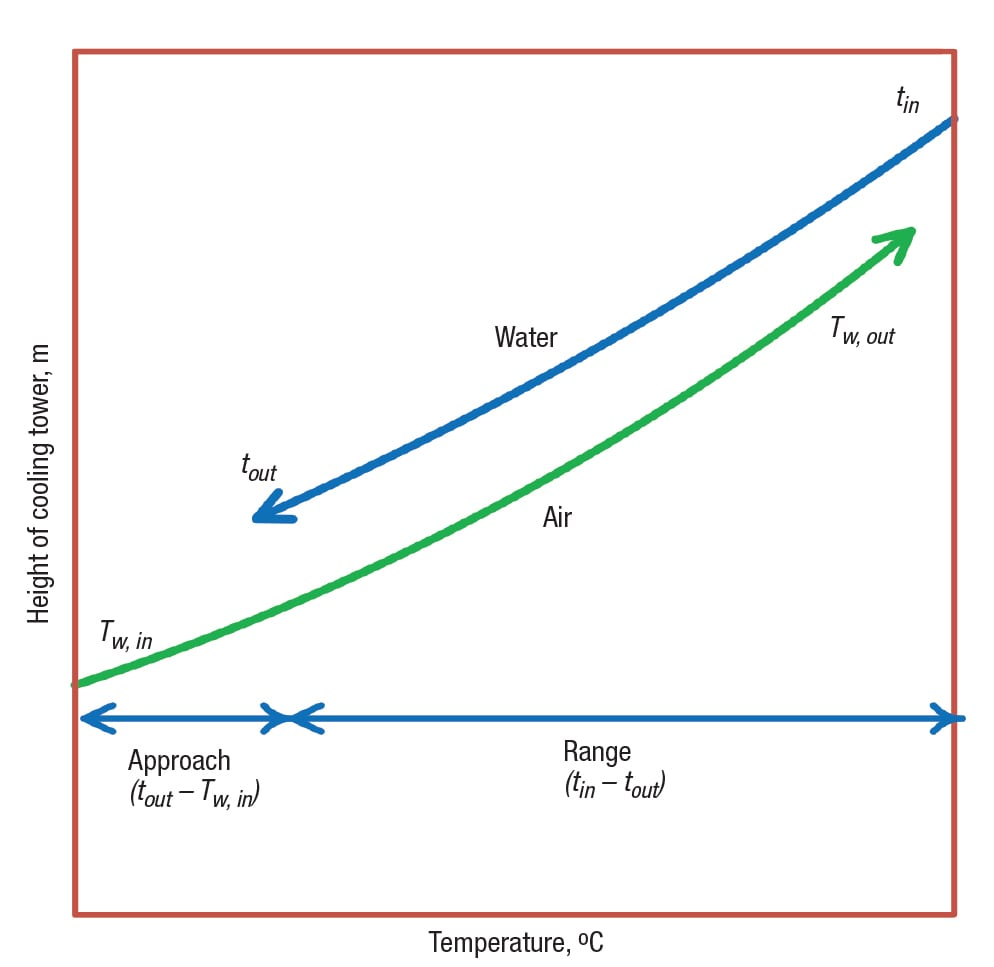FIGURE 1. Shown here is the typical variation of the water temperature and the wet-bulb temperature of the air stream as the hot water inlet stream flows down from the top of the cooling tower and the air stream flows upward along the height of the cooling tower

The cooled water is collected in the sump (or basin) of the cooling tower, and it is typically pumped to the plant as the cooling-water-supply (CWS) stream. After extracting heat from the process units, this stream is returned to the cooling tower, as the cooling-water-return (CWR) stream. The heat load extracted from the process unit is finally released to the environment in the cooling tower. A cooling tower is designed to remove the total heat load that is extracted from the plant by reducing the CWR temperature to the CWS temperature.

## Case study

The CWR line from the process unit enters an industrial cooling tower at 45°C and leaves at 33°C, as shown in Figure 2. The tower has three cells, each operating at 2,500 m 3 /h of water flow. The total flow 7,500 m 3 /h is measured at the CWR line. The dry-bulb temperature and wet-bulb temperature of the inlet air are measured as 30.3°C and 29°C, respectively. The dry-bulb temperature of the exit air is 41.5°C, and it is assumed to be 100% saturated. This case study is aimed at calculating the unknown variables — that is, evaporation loss, air flow through the tower, blowdown flow, and the required makeup water flow. First, the important parameters — approach, range and efficiency — are detailed.

Approach. The approach is defined as the difference between the water temperature at the tower outlet ( t out) and the wet-bulb temperature of the inlet air ( T w,in). The approach represents the cooling tower capability. In general, the larger the tower, the smaller is the approach. In this case study, the approach is 4°C.

Theoretically, the extent of maximum possible cooling that could be achieved through a cooling tower would be to produce a stream that is at the wet-bulb temperature of the ambient air. However, to achieve this theoretical maximum, the tower would need to have infinite height. So the practical limit of the CWS temperature is generally considered to be 4°C above the wet-bulb temperature of ambient air. For design purposes, the worst scenario — that is, the summer season wet-bulb temperature — needs to be considered.

Range (ΔT). The range is the difference between the water temperatures at the inlet and the outlet of the cooling tower (t in – t out). In this case, the range is 12°C. The range does not represent the cooling tower capability; rather, the range is based on the cooling-water circulation flowrate ( L in), and the sum of the heat loads taken from the heat exchangers in the process unit ( Q), and it is not related to the size or capability of the cooling tower. On the other hand, an increase in range will cause an increase in approach, if all other conditions are not changed. The range is shown in Equation (1):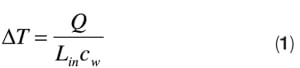Cooling tower efficiency (). The cooling tower efficiency is the ratio of actual cooling (range) to the theoretically possible maximum cooling (that is, when the approach is zero), as shown in Equation (2):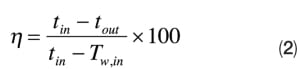Theoretically, an approach of zero means the tower is 100% efficient. Industrial cooling towers typically have an approach temperature between 4° and 8.5°C, and an efficiency between 70 and 75% [ 2]; in this case, the efficiency is 75%.

## Evaporation loss and air needs

Method 1. The evaporation loss and air flow requirement through the tower can be evaluated by solving the mass and energy balance equations simultaneously.

Use Equation (3) to apply a mass balance for the entire section of tower. As shown in Equation (3), the amount of water evaporated ( e L) in the down-pouring liquid is the difference between the inlet liquid flow ( L in) and the sum of the outlet liquid flow (L out) and the drift loss ( d L). It is equal to the difference of moisture content of air across the tower.

Where:

G’ = the quantity of dry air flow (which remains the same at the inlet and outlet air streams), kg of dry air

Y = absolute humidity, kg water/kg dry air/h

The subscripts in and out refer to the entry and exit locations.

The overall energy balance is given by Equation (4):

Where:

h = the liquid enthalpy, kJ/kg water

H = the moist air enthalpy, kJ/kg dry air

Substituting L out from Equation (3) into Equation (4), and assuming the enthalpy of the drift water h d is h out, and simplifying Equation (4), one gets Equation (5):

Solving both mass and heat balance equations [Equations (3) and (5)] simultaneously, the evaporation loss ( e L) and the dry-air requirement ( G’) are estimated as 132,000 kg/h and G’ = 4,699,850 kg dry air/h, respectively.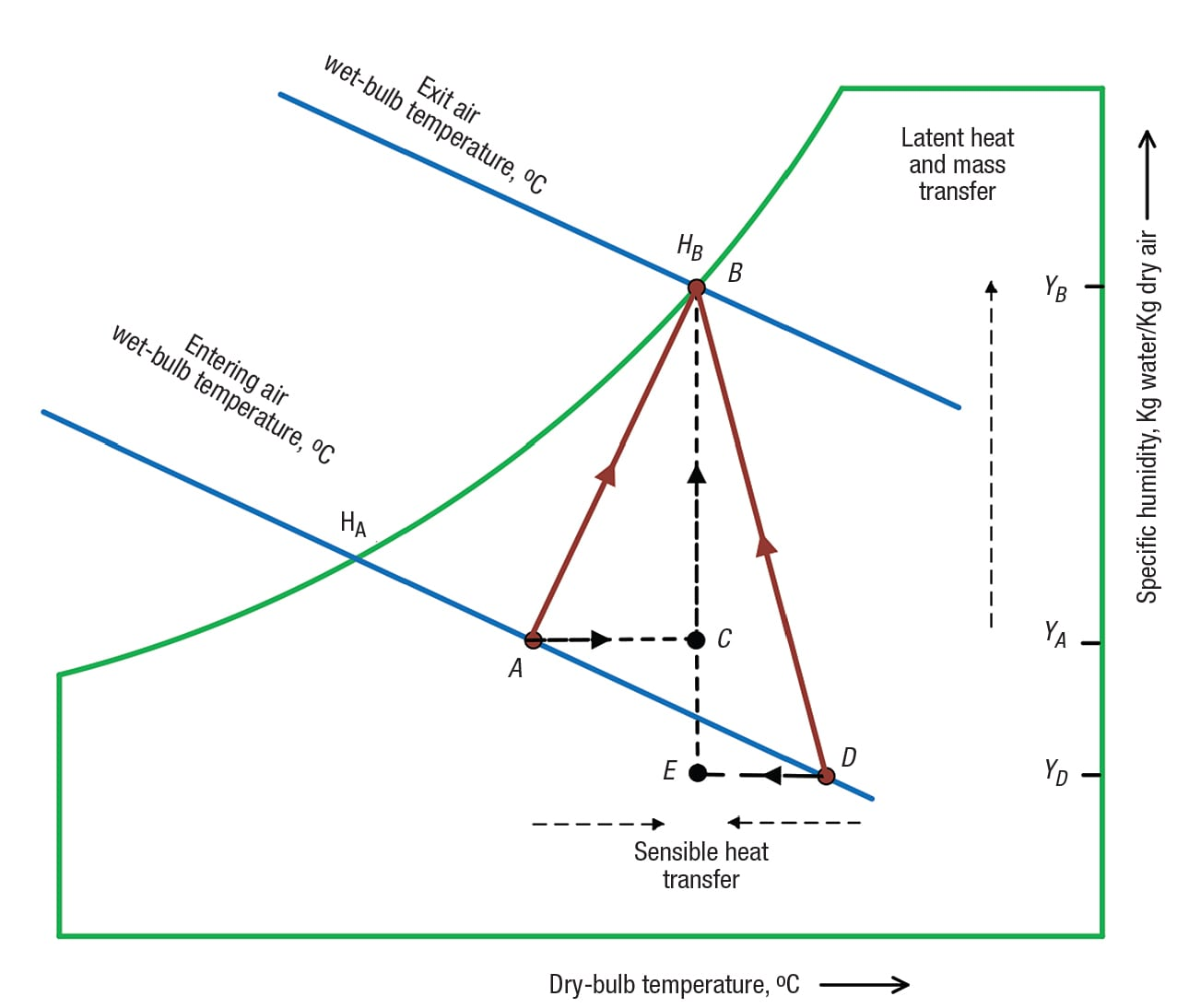FIGURE 3. In this psychrometric chart, the relevant process condition from the case history is marked as vector AB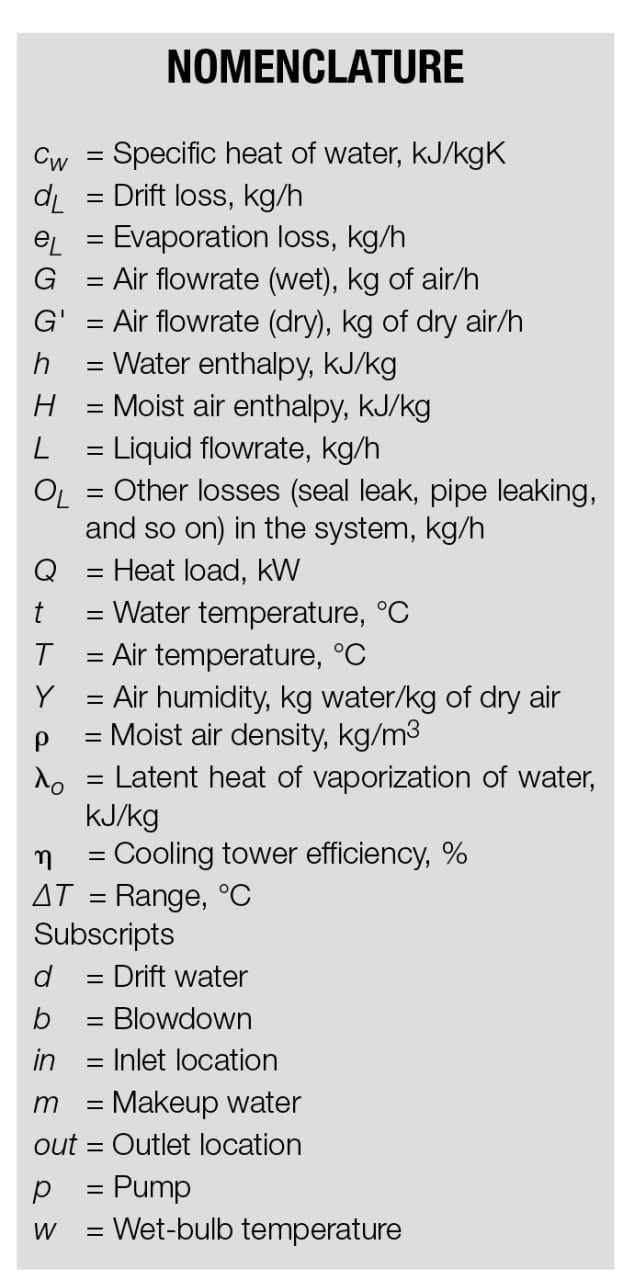Method 2. Depending on the temperature of the inlet air (whether it is hot or cold), the air can be either heated or cooled as it travels along the height of a cooling tower. In the psychrometric chart shown inFigure 3, the entering condition of the air is denoted by point A, and the exit air (which is completely saturated with water) is denoted by point B. The enthalpy difference of dry air is ( H A –H B). The vector AB is the sum of the two components. The horizontal component AC represents the sensible heating of air, and the vertical component CB is the latent heating of air. In a cooling tower, it is also possible to cool the air if the inlet air condition is at D[ 3]. At point D, the air is hot and dry, when compared to the air at point A.

The component DE is the sensible air cooling, and the component EB is the latent heating of air. The net heat received by the air is the difference between the latent air heating and the sensible air cooling.

In the case of the AB process, the dry-bulb temperature of the air is increased at the exit — that is, the exit air becomes hot compared to the inlet air. But in the case of DB, the dry-bulb temperature of air is decreased and thus the air is cooled at the exit. In both cases, the wet-bulb temperature of the exit air will always be increased compared with that of the inlet air. So, the water flowing through the cooling tower can be cooled by unsaturated air, irrespective of whether the air is hot or cold.

In this case study, from the field measurements of DBT and WBT, the psychrometric properties, such as absolute humidity, saturation humidity and moist air enthalpy for the inlet air and the outlet air, could be evaluated. The inlet air is marked as point A, and the outlet air is marked as point B in the psychrometric chart. Another hypothetical point C is marked in such a way that it has a dry-bulb temperature similar to point B and absolute humidity similar to point A. It must be noted that the point C is a hypothetical and does not correspond to any location in the cooling tower; the point C is marked on the chart to see the horizontal and vertical component of vector AB. Moist air enthalpy for point C is calculated.

The total heat gained by the air ( H B –H A) has two components: the latent heat transfer ( H B –H C), and the sensible heat transfer ( H C –H A). The ratio of latent heat transferred ( e Lo) to the total heat released from the water side is shown by on the left in the Equation (6), and this expression is numerically equal to the ratio of latent heat added to the dry air to the total heat gained by the air, which is shown on the right side in the Equation (6):

From Equation (6), e L is calculated as 132,000 kg/h. It is to be noted that in this method, the dry air flow ( G’) is not required. Once e L is evaluated, G’ is estimated from the mass balance equation [(Equation (3)]. The split of latent heat transfer and sensible air heating in this case is about 85% and 15%, respectively.

## Makeup water and blowdown

Makeup water ( L m) is added to the sump to compensate for the water losses in the circuit. The water losses include evaporation loss ( e L), drift loss ( d L), blowdown ( L b), and other leakage losses ( O L) in the system, such as losses from the pump seal, piping leak, washdown water and filter backwash.

Drift loss.Small droplets that are entrained by the upward-flowing air stream are collected in a mist eliminator, where they accumulate to form larger drops that are eventually returned to the fill. In general, very little water in the form of droplets is carried along with the air, but those droplets do results in water loss, called drift loss or windage loss. This drift water typically contains dissolved solids and may cause stain, corrosion or damage to nearby buildings and structures. Drift loss is usually about 0.1–0.3% of the circulation water rate ( L in).

To compensate for the evaporation loss and drift loss, additional makeup water is added. Since the makeup water typically contains dissolved solids, these solids are typically left behind in the sump water as the water evaporates in the cooling tower. Meanwhile, since the cooling water is a very effective air scrubber, dust and debris present in the up-flowing air is washed out by down-pouring water and collects in the sump. As solids accumulate in the sump, they increase the potential for scale corrosion and biological fouling in the cooling-water circuit. By taking small amounts of water continuously from the cooling tower circuit (blowdown), the concentration of dissolved solids in the cooling water can be reduced below the upper limit of the acceptable range, in order to meet the cooling-water quality specification of the plant.

Blowdown. There are two ways to remove the blowdown — as hot blowdown and cold blowdown (Figure 2). Hot blowdown refers to the continuous removal of water in the cooling-water-return line to the effluent. Since the water is hot at this location, hot blowdown may not be acceptable in some applications due to potential environmental impact; in other cases, it is desired, since it reduces throughput to the cooling tower and increases overall cooling performance.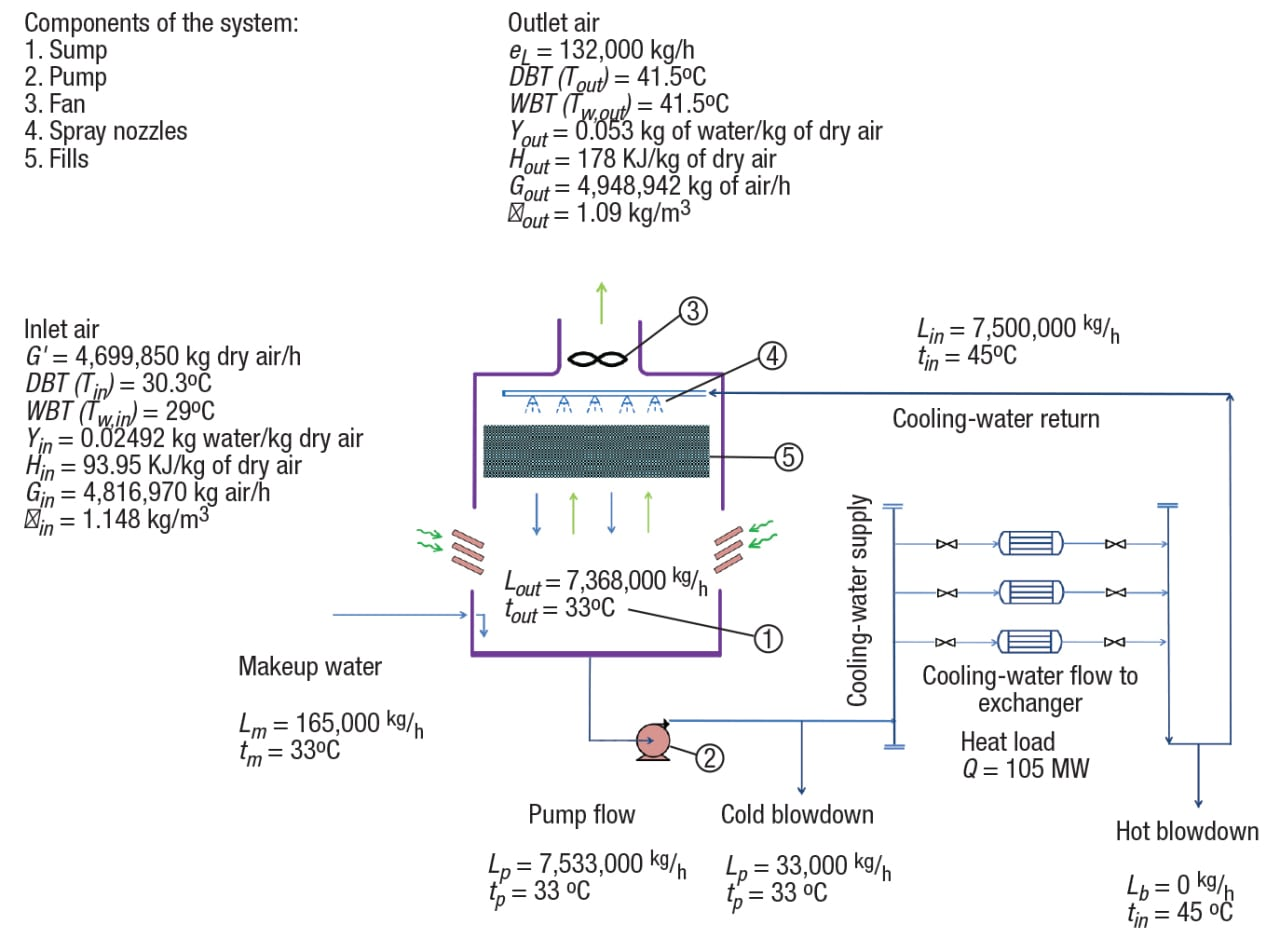FIGURE 2. This schematic diagram depicts the parameters of the case study cooling tower system.
Note: All three cells and three fans are lumped together and shown as a single unit

Cold blowdown refers to the continuous removal of water from the cooling-water pump outlet to the effluent [ 4]. Drift loss and any leakage loss from the system are also considered as blowdown, since these streams contain dissolved solids (but such losses are unintentional).

The amount of water blowdown is established by calculating the cycle of concentration ( CC), which is defined as the ratio between the amount of solids dissolved (mostly chlorides) in the blowdown and in the makeup water, using Equation (8):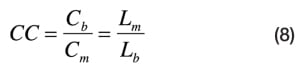Assuming drift loss and leakage losses are negligible, and solving the water-balance shown below in Equation (9):

The dissolved-solids balance shown below in Equation (10), the blowdown is calculated using Equation (11):

Further, the amount of makeup water needed is estimated, including drift, using Equation (12):

The required makeup water mainly depends on evaporation loss and the CC calculated above. It is to be noted from Equation (11) that the minimum value of CC to be considered is 2, which requires blowdown to be at the same amount of water as the amount lost in evaporation. Any attempt to reduce the CC below 2 results in a significant amount of makeup water, as shown in Figure 4.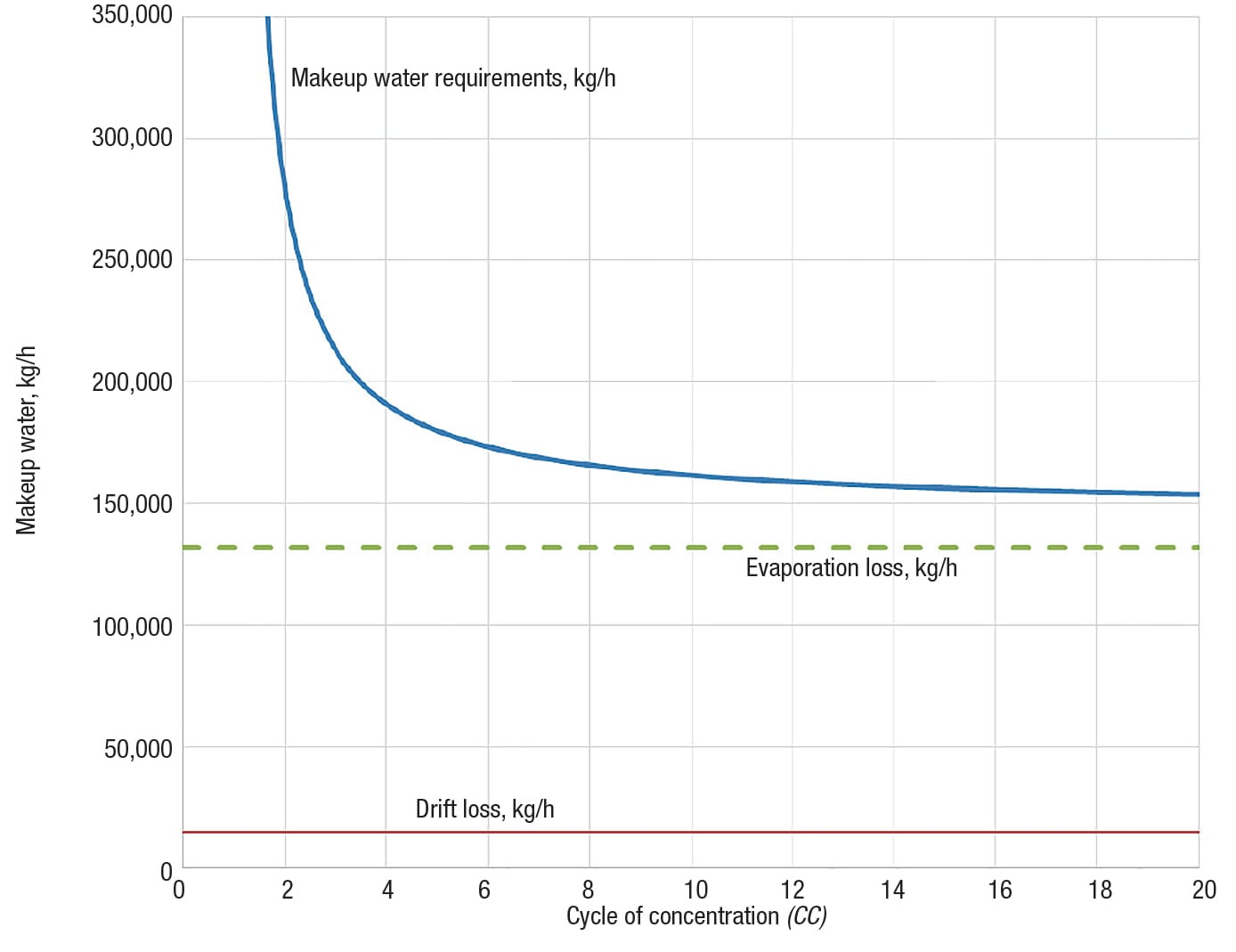FIGURE 4. Makeup water requirements versus the cycle of concentration are shown here

Higher CCmeans that C m tends to zero (indicating good quality of the makeup water). But, this is achieved at the cost of water treatment of the source water. A typical cycle of concentration ( CC= 5) is considered in this case study for the optimum requirement. Based on the evaporation loss and cycle of concentration, cold blowdown and makeup water are calculated using Equation (11) and Equation (12), as 33,000 kg/h, and 165,000 kg/h, respectively. Further, assuming 0.2% drift loss and no system leak, makeup water needs to be considered as 180,000 kg/h. n

Edited by Suzanne Shelley

## References

1. American Petroleum Institute, Programme in Learning Operating Techniques — Cooling Towers, 1995.

2. Huchler, L., Cooling Towers, Part 2: Operating, Monitoring and Maintaining, Chemical Engineering Progress, Oct. 2009.

3. American Society of Heating, Refrigerating and Air Conditioning Engineers, “ASHRAE Handbook,” Chapter 39 — HVAC System and Equipment, 2008.

4. Smith, R., ““Chemical Process Design and Integration,” John Wiley & Sons Ltd., 2005.

## AuthorUthirapathi Vengateson is a senior process design engineer at National Petrochemical Co. in Yanbu, Saudi Arabia (Phone: +966 534878029; Email: [email protected], [email protected]). For 17 years, Vengateson has been involved in process engineering design, research and development, and commissioning of chemical and petrochemical plants. Prior to this, he worked in Lurgi India Company Ltd. in New Delhi, India. Vengateson earned a bachelor’s degree (B.Tech.) in chemical engineering from Madras University, a master’s degree in petroleum refining and petrochemicals from Anna University, and a Ph.D. in chemical engineering from Indian Institute of Technology, New Delhi, India.